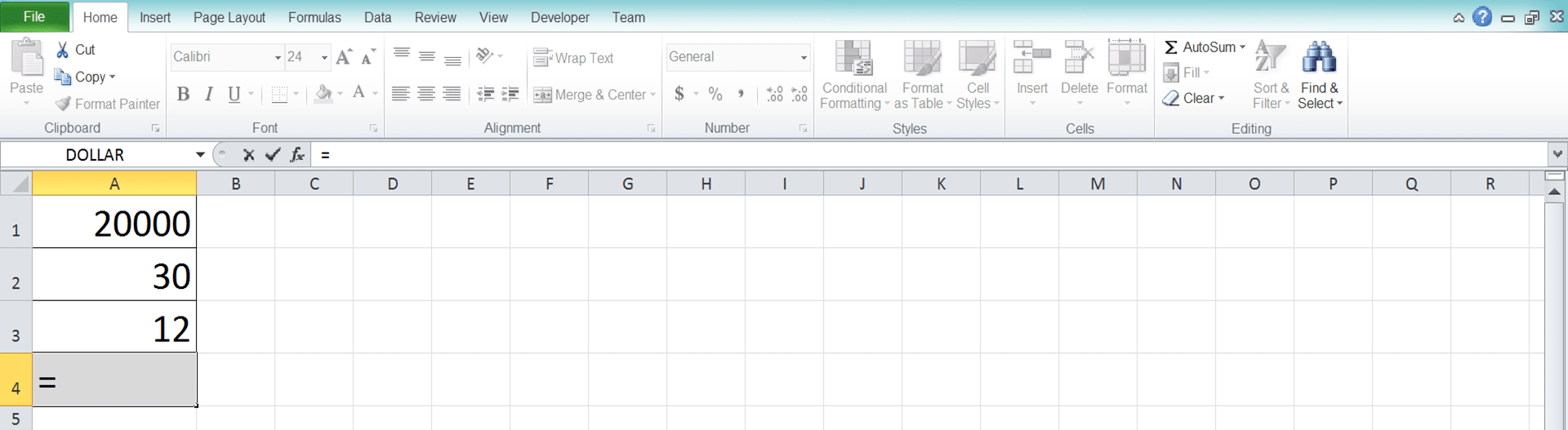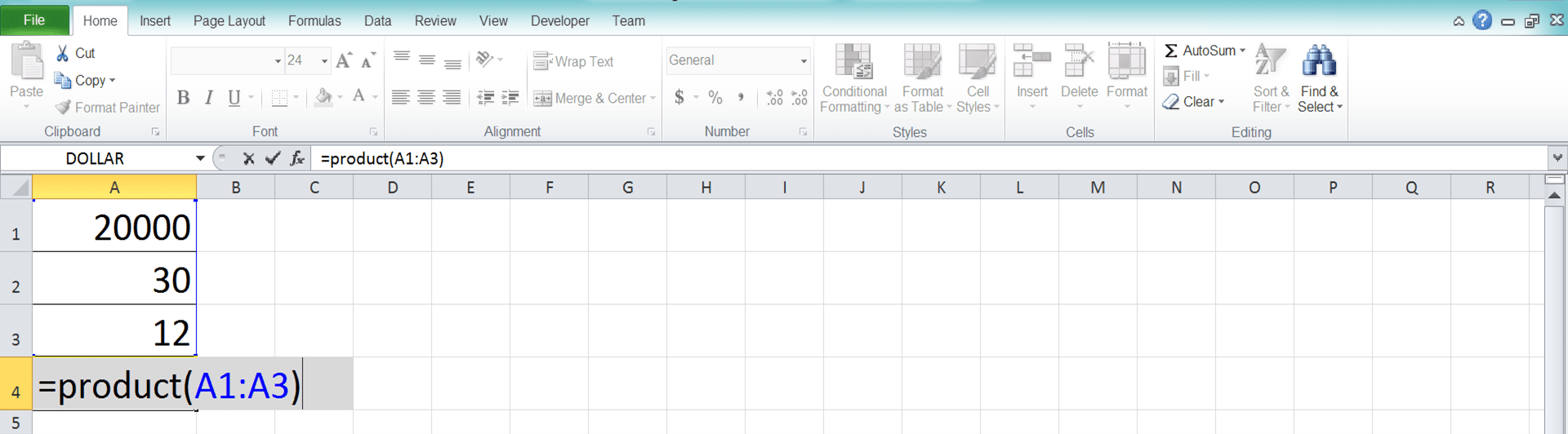How to Use the PRODUCT Formula in Excel: Functions, Examples, and How to Write It - Compute Expert

# How to Use the PRODUCT Formula in Excel: Functions, Examples, and How to Write It

Home >> Excel Tutorials from Compute Expert >> Excel Formulas List >> How to Use the PRODUCT Formula in Excel: Functions, Examples, and How to Write It

In this tutorial, you will learn how to use the PRODUCT formula in excel completely.

When working with numbers in excel, we often need to multiply our numbers to get the result that we want. If you need to multiply many numbers, you can use PRODUCT to get your multiplication result easily and fast.

Want to know more about PRODUCT and understand the way to use this formula properly in excel? Read this tutorial until its last part!

Disclaimer: This post may contain affiliate links from which we earn commission from qualifying purchases/actions at no additional cost for you. Learn more

## What is the PRODUCT Formula in Excel?

PRODUCT is an excel formula that helps you to get the multiplication result of your numbers.

## PRODUCT Function in Excel

You can use PRODUCT to multiply your numbers easily and fast in excel. We usually use this formula if we need to perform multiplication on many numbers in a cell range.

## PRODUCT Result

The PRODUCT result is a number that represents the multiplication result of the numbers we input into it.

## Excel Version from Which We Can Start Using PRODUCT

We can start using the PRODUCT formula in excel since excel 2003.

## The Way to Write It and Its Inputs

Here is the general writing form of PRODUCT in excel.

= PRODUCT ( number1 , number2 , … )

When you use PRODUCT, you only need to input the numbers you want to multiply into it. These numbers input can be in the form of a number, cell, or cell range. Don’t forget to write comma signs ( , ) in between the inputs you give to PRODUCT.

You can input up to 255 different inputs to PRODUCT.

## Example of Its Usage and Result

Here is an implementation example of PRODUCT in excel.As you can see in the example, we can easily get our numbers multiplication result by using PRODUCT. Just input the numbers we want to multiply into the PRODUCT formula that we write.

The example (the fourth and fifth rows) also shows that PRODUCT ignores things such as empty cells, texts, or logic values in its multiplication process.

## Writing Steps

Need guidance when you write your PRODUCT formula in excel? Take a look at the PRODUCT formula writing steps below!

1. Type an equal sign ( = ) in the cell where you want to put your multiplication result2. Type PRODUCT (can be with large and small letters) and an open bracket sign after =3. Input the numbers you want to multiply. You can input them directly or through cells or cell ranges. Place comma signs between all the inputs you give to PRODUCT4. Type a close bracket sign after you have inputted all the numbers you want to multiply5. Press Enter
6. Done!## Why Does PRODUCT Give Me a Wrong/Error Result? Possible Reasons and Solutions

The PRODUCT formula you write gives you a wrong multiplication result or even an error? Many things can cause this to happen. Here are the three of them which are probably the most possible reasons for that to happen and their solution.

• Reason: You have number text in your PRODUCT input
Solution: PRODUCT ignores numbers with text data type in its multiplication calculation. Retype your number or use the VALUE formula to convert your number text into a real number

• Reason: You have errors in the inputs you give to PRODUCT
Solution: Even just one error (most probably in the cell range you input into your PRODUCT) can make your PRODUCT result becomes an error too. Delete/modify the error values or use IFERROR to anticipate the errors if they come from the formulas you write

• Reason: You have date/time data in your PRODUCT input
Solution: Date/time data is a number in its real form in excel. Thus, it can mess up your PRODUCT calculation if it becomes its input. Delete the date/time data or take them out from your PRODUCT inputs so you can get the correct multiplication result

Check again your PRODUCT formula writing (especially its inputs) for those points above. We hope they can help you get the right multiplication result from your PRODUCT!

## Exercise

After you have learned how to use the PRODUCT formula in excel, now let’s do an exercise!

### Instructions

Use PRODUCT to do all the instructions below.
1. Get the multiplication result from the numbers in cell A2 until C8!
2. Get the multiplication result from the numbers in cell E5 until G9!
3. Get the multiplication result from the numbers in cell H7 until J9!

We usually use PRODUCT only if we need to multiply many numbers in a cell range. If you just multiply few numbers, you may want to multiply them manually (using the star symbol ( * )) since it should be simpler that way.

Related tutorials you should learn:

Get updated excel info from Compute Expert by registering your email. It's free!